Describing motion along a line

Start this free course now. Just create an account and sign in. Enrol and complete the course for a free statement of participation or digital badge if available.

Free course

# 5.4 Drop-towers revisited

In Section 1 we described how research into near weightless conditions can be carried out on Earth by using a drop-tower or a drop-shaft (Figure 41). We are now in a position to examine drop-shafts in more detail (Example 3).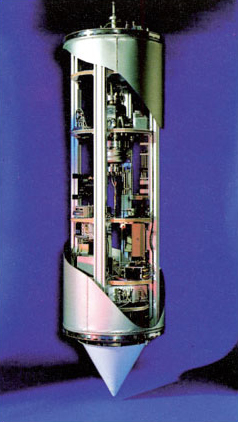Figure 41: The test vehicle at the Bremen drop-tower

## Example 3

Consider a vertical shaft of total length 700 m, with free fall taking place for the first 500 m and constant deceleration for the final 200 m. Work out:

1. The time to fall the first 500 m. (This would be the time during which near weightless experiments could be done.)

2. The velocity at the end of the first 500 m.

3. The acceleration needed to reduce the velocity to zero at the bottom of the shaft.

It is convenient to split the calculations into three corresponding parts.

Part 1: Finding the time to fall the first 500 m

When carrying out calculations it is always wise to choose a coordinate system that fits the problem. In this case it seems natural to choose an x﻿-﻿axis that points down the shaft, so that downward displacements and velocities will be positive and the acceleration due to gravity will be a﻿x = + g. It is also sensible to use the equations that lead to the least amount of work. In this case, we know only the initial velocity (assuming the object is initially at rest), the acceleration (g) and the final displacement (500 m). So, in order to work out the time to fall, the best approach is to use Equation 28a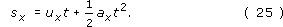with s﻿x﻿ = 500 m, u﻿x = 0 m s﻿−﻿1 and a﻿x = g = 9.81 m s﻿−﻿2. Setting u﻿x = 0 m s﻿−1 and rearranging Equation 28a we obtain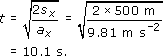Part 2: Finding the velocity at the end of the first 500 m

To calculate the velocity after a free fall of 500 m, there are two convenient methods. We could either use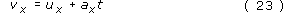with ux = 0 m s−1, ax = g = 9.81 m s−2 and t = 10.1 s, or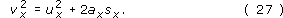with ux = 0 m s−1, ax = g = 9.81 m s−2 and sx = 500 m.

We choose the second method since it uses only values that we have been given rather than ones we have calculated. (It is always possible that we made some slip in our calculation.) So using Equation 28c with u﻿x = 0 m s﻿−﻿1, we obtain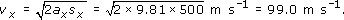Part 3: Finding the acceleration over the final 200 m

To find the uniform acceleration necessary to bring the object to rest at the bottom of the shaft we can again make use of Equation 28c. This time, the quantity ax is what we want to calculate and the known parameters are v﻿x = 0 m s﻿−﻿1, u﻿x = 99.0 m s−1 and s﻿x = 200 m. Substituting v﻿﻿x = 0 m s﻿−﻿1 and rearranging Equation 28c gives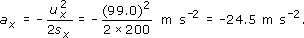## Question 24

Consider the following proposal to roughly double the period of weightlessness in the 140 m Bremen drop-tower. The basic idea is that the drop-vehicle should be launched from the bottom of the tower with just the right velocity to enable it to reach the top of the tower with zero velocity. If the vehicle is uniformly accelerated over the first 10 m to give it the necessary upward velocity it can then rise freely for the remaining 130 m, pass through its highest point, and fall freely for another 130 m before being brought to rest over the final 10 m of its descent. Using a coordinate system in which the upward direction is positive, answer the following questions.

(a) What must be the launch velocity of the vehicle if it is to freely rise 130 m?

(b) What constant total acceleration must be applied over the first 10 m if the vehicle is to attain the required launch velocity? How long will this last?

(c) How long will the vehicle actually spend in free motion?

(d) What will be the acceleration of the vehicle when at its highest point?

(e) What will be the displacement of the vehicle from its starting point when it returns to that point at the end of the trip, and through what distance will it have travelled during the round trip?

(f) Sketch a rough acceleration-time graph for the entire motion, and briefly comment on the feasibility of the whole proposal.

(a) Using a coordinate system in which up is the positive direction means that the acceleration due to gravity will be negative, that is ax = −g. With vx = 0 m s−1 and sx = 130 m, the initial velocity will be given (as in Question 22) by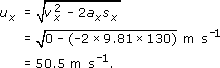(b) In this case we know ux = 0 m s−1, vx = 50.5 m s−1 and sx = 10.0 m. (Note that what was an initial velocity in the last part of the question has become a final velocity in this part.) Rearranging Equation 28c and substituting the given values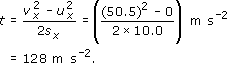To find the duration of the acceleration rearrange Equation 28b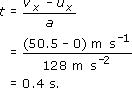(c) The time in free motion is given by Equation 26 with ux = 50.5 m s−1, vx = −50.5 m s−1 and ax = −9.81 m s−2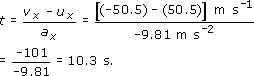(Pay attention to signs here, remember that up is positive so the initial velocity will be positive, but the final velocity will be negative.)

(d) When at its highest point the acceleration of the vehicle will be ax = −g. (The fact that the vehicle's velocity is momentarily zero as it passes through the highest point does not affect the (constant) acceleration.)

(e) When the particle returns to its starting point its displacement from that point will be zero. The distance travelled, however, will be twice the height of the tower, 280 m.

(f) The acceleration-time graph is given in Figure 42. Note that the initial and final accelerations are both positive because both increase the vehicle's velocity, even though the final acceleration reduces the vehicle's speed. (This subtlety concerning acceleration was discussed in Section 4.2.) The plan is feasible. In fact a similar system is in use at various drop-towers and drop-shafts.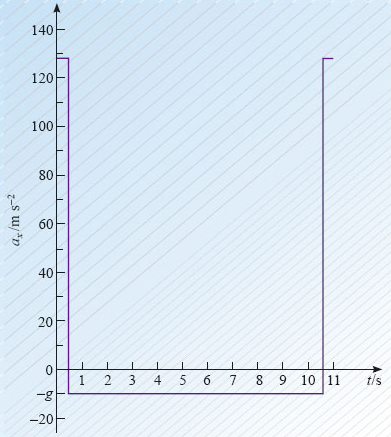Figure 42: The acceleration-time graph for Question 24(f)
S207_2